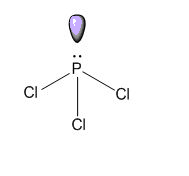# What kind of a molecule in $\text{PC}{{\text{l}}_{\text{3}}}$?A.) IonicB.) Polar covalentC.) Non- polar covalentD.) CoordinateVerified
174.9k+ views
Hint: Bond polarity is determined by electronegativity difference between the atoms, while polarity of a molecule is determined by dipole moment.
$\text{PC}{{\text{l}}_{\text{3}}}$ Shows structural similarity with $\text{N}{{\text{H}}_{\text{3}}}$ and both the molecule are polar molecule.

Complete Solution :
Dipole moment is a vector quantity, and the dipole moment of a polar molecule depends on its geometry. A symmetrical molecule or having a regular geometry, is non- polar even though it contains polar bonds because in a symmetrical bond dipole of all the bonds cancel out by each other. Only the molecule which has an asymmetrical shape has a dipole moment.
- In $\text{PC}{{\text{l}}_{\text{3}}}$, the central phosphorus atom is attached with three chlorine molecule by covalent bond and, has a lone pair. $\text{PC}{{\text{l}}_{\text{3}}}$ has distorted structure due to the presence of lone pairs of electrons.(A) $\text{PC}{{\text{l}}_{\text{3}}}$ Is contains three covalent bond which are formed by equal sharing of electron in between phosphorus atom and chlorine atom, hence it’s a covalent molecule.
(B) $\text{PC}{{\text{l}}_{\text{3}}}$ Molecule is an asymmetrical molecule, and has a net dipole moment. So, this is a polar covalent molecule.
(C) Due to the absence of molecular symmetry, $\text{PC}{{\text{l}}_{\text{3}}}$ has a net dipole moment, so it is a polar molecule.
(D) Coordinate bonds are formed by unequal sharing of electrons in between the two atoms. In $\text{PC}{{\text{l}}_{\text{3}}}$ only covalent bonds are present hence it is a covalent molecule.
So, the correct answer is “Option B”.

Note: - Dipole moment in a polar covalent bond is equal to the product of induced charge on covalently bonded atoms and their intermolecular distance.
-Dipole moment depends upon the symmetry of the molecule, and symmetry of the molecule is mainly determined by the presence of lone pairs and bond pairs of central atoms.